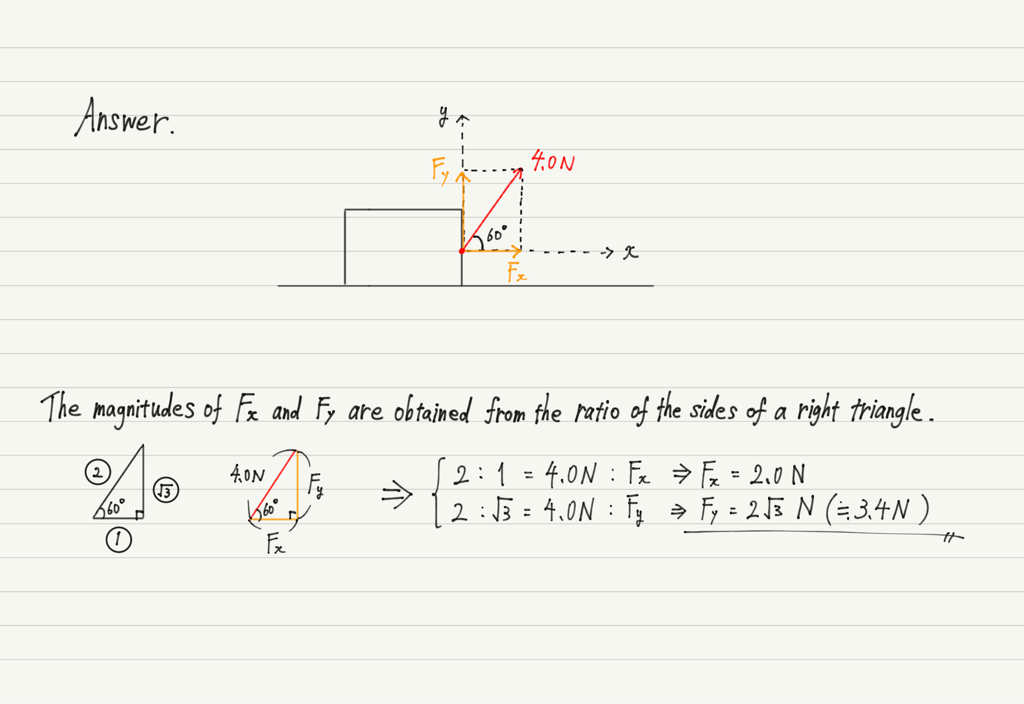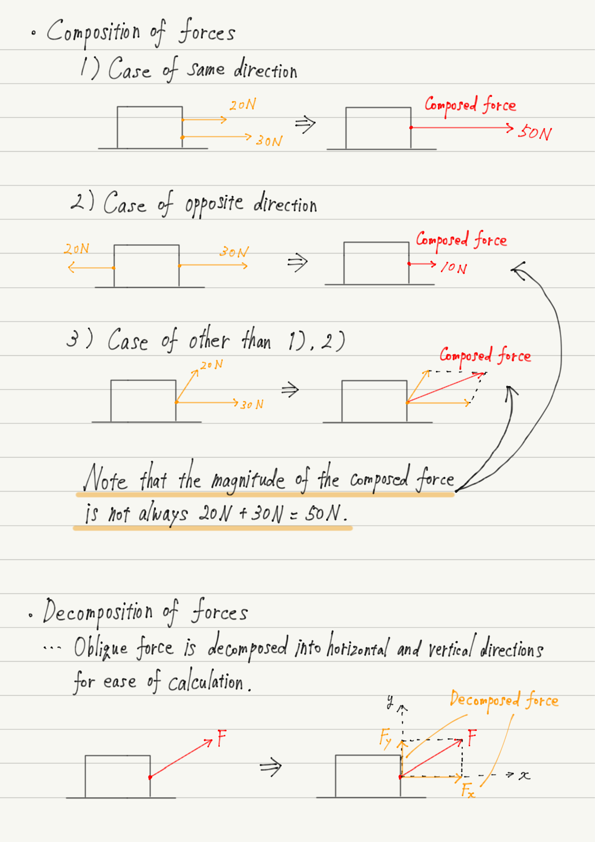# Composition and Decomposition of Forces

In the previous lectures, we have finished introducing the main forces appearing in mechanics.
From this point on, the main theme will be to investigate the relationship between the motion of objects and forces. To prepare for that theme, let’s study the calculation of forces.

## Composition of forces

In reality, there is not always a single force acting on an object. Rather, it is more common for there to be multiple forces.
When multiple forces act on an object, it is easier to treat them as a single force instead of considering them separately.

Now let’s look specifically at the method of force composition.
The first case is when the two forces point in the same or opposite directions.As shown in the figure, it is simple to add or subtract the magnitudes of the forces.

Then, what about the following cases?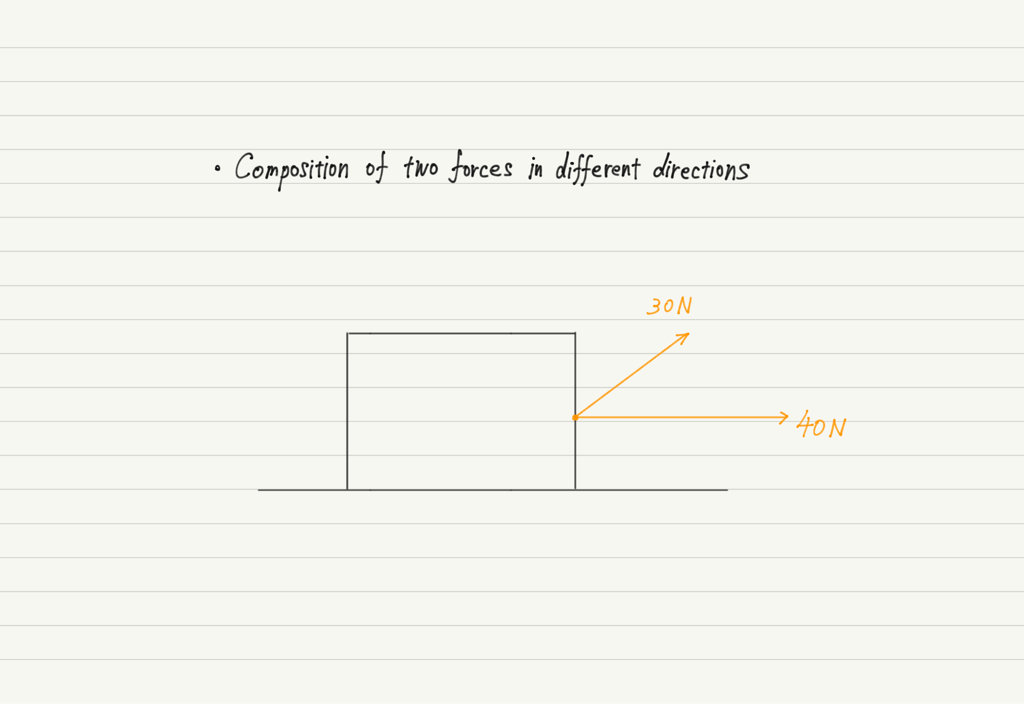30 N force composed with 40 N force, so 70 N?

No, that is not true.

For normal addition, 1+1=2, but in the case of force composition, 1 N force and 1 N force do not necessarily add up to 2 N!

Recall the previous example. If two forces of 1N act in the same direction, the total force is 2N, but if they act in opposite directions, they cancel each other out and become 0.

Thus, we can see that “Direction” is important in force composition. In this case, the directions of the two forces are not aligned, so it is wrong to add the magnitudes as they are!

When combining forces of different directions, parallelograms are used.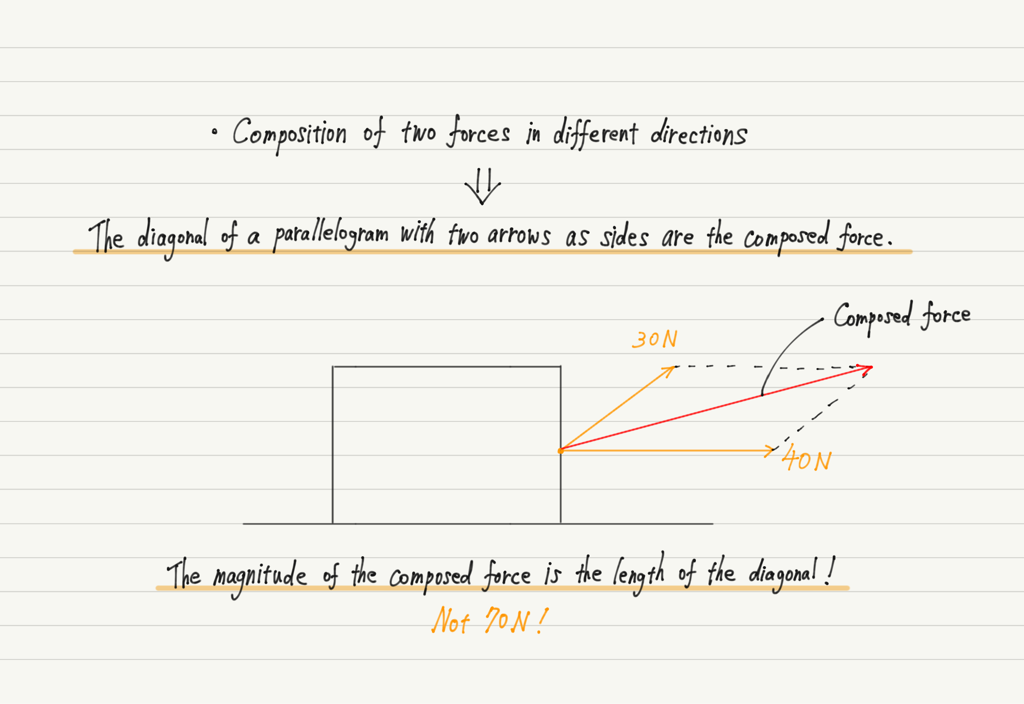To summarize, let’s try one exercise!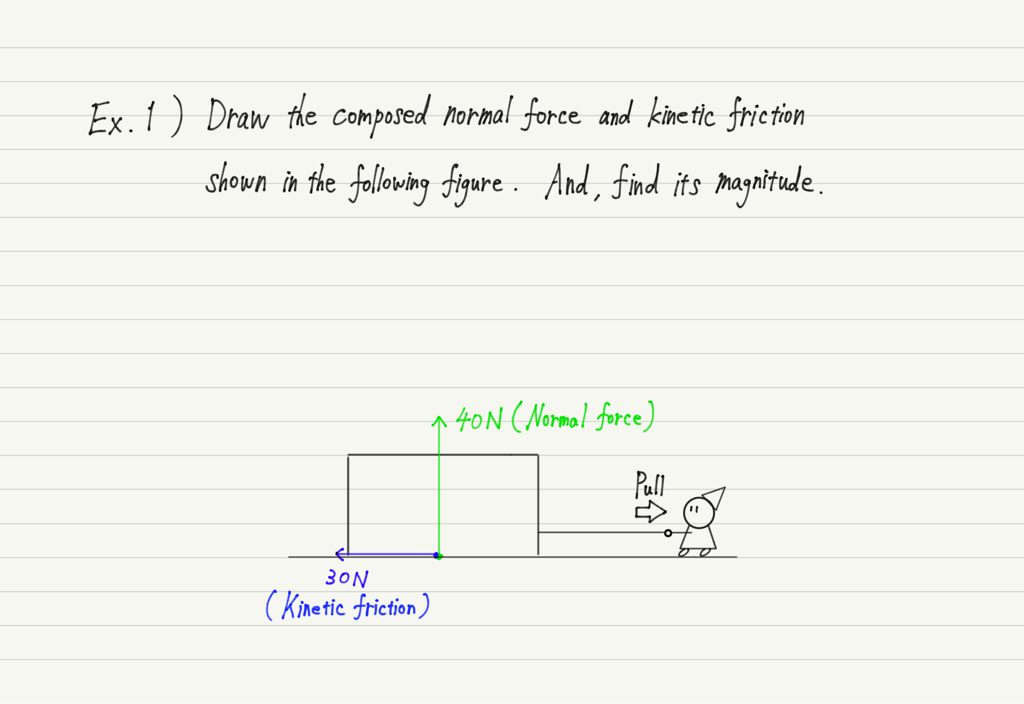Answers are below, but please try to solve it by yourself first.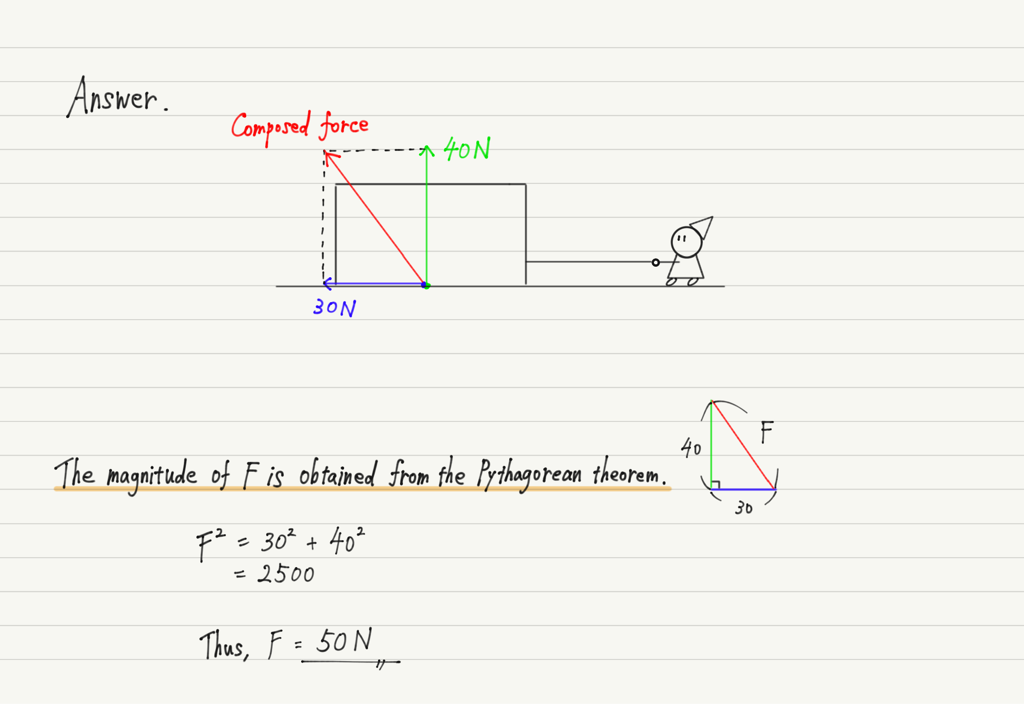Force composition is possible even when there are three or more forces. First, A and B are combined, then the combined force and C are combined, and so on, one by one.

## Decomposition of forces

If combining two forces into one is force composition, then decomposing one force into two is force decomposition.
It is not enough to decompose everything, since decomposing a force increases its number.

There is only one situation in which power must be decomposed.
This is the case when a “oblique force” is acting on an object.

As we will see a little further down the road, when investigating the motion of an object, it is fundamental to “divide it into Vertical and Horizontal directions”.
If there is a diagonal force at that time, it will not work well, so we have to decompose the force.

See the figure below for the specific method of force decomposition.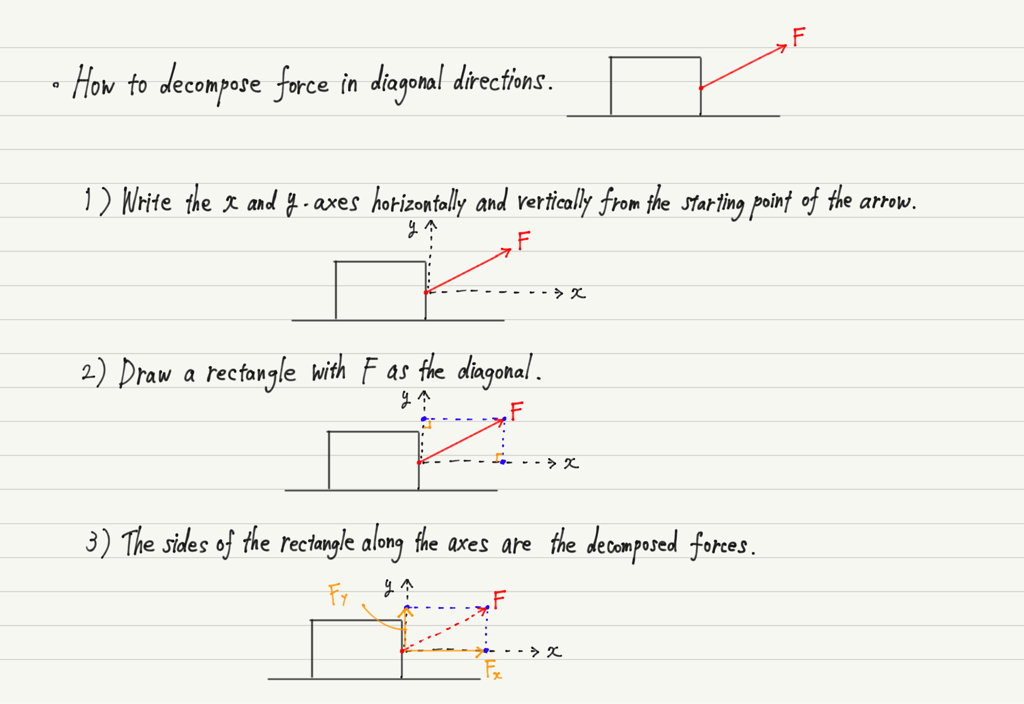This shows that force decomposition is the opposite of force composition.
Now, let’s do exercises!